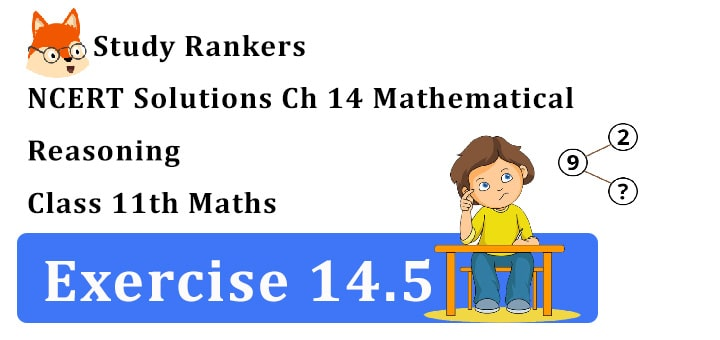>

## NCERT Solutions for Class 11 Maths Chapter 14 Mathematical Reasoning Exercise 14.5

Chapter 14 Mathematical Reasoning Exercise 14.5 Class 11 Maths NCERT Solutions are very useful if you want to improve your problem solving skills. Here, you will find NCERT Solutions for Class 11 Maths which are prepared by Studyrankers expert that will help you in completing your homework. By practicing NCERT Questions, you will get to know about important points provided in the chapter.1. Show that the statement p:
"If x is real number such that x 3 + 4x = 0 then x = 0" is true by
(i) Direct method
(iii) Method of contrapositive.

(i) Direct method.
x 3 + 4x = 0 or x(x 2 + 4) = 0
=> x 2 + 4 ≠ 0, x ∈ R hence, x = 0
Let x ≠ 0 and let it be x = p, p∈ R, p is a root of x 3 + 4x = 0
∴ p 3 + 4p = 0 ∴ p(p2 + 4) = 0
p ≠ 0 Also p2 + 4 0 => p = 0
(iii) Contrapositive. q is not true
=> Let x = 0 is not true
=> let x = p ≠ 0 ∴ p 3 + 4p = 0,
p being the root of x 2 + 4 = 0
or p(p2 + 4) = 0, Now, p = 0 Also p2 + 4 ≠ 0 => p(p 2 + 4) ≠ 0 if p is not true
∴ x = 0 is the root of x 3 + 4x = 0.

2. Show that the statement “For any real numbers a and b, a 2 = b 2 implies that a = b” is not true by giving a counter-example.

Let a = 1, b = –1
and a 2 = b 2 but a ≠ b
Thus we observe that the given statement is not true.

3. Show that the following statement is true by the method of contrapositive.
p: If x is an integer and x2 is even then x is also even.

Let x is not even -i.e. x = 2n + 1
∴ x2 = (2n + 1)2 = 4n 2 + 4n + 1
= 4(n 2 + n) + 1
4(x 2 + x) + 1 is odd. i.e. "If q is not true then p is not true" is proved
Hence, the given statement is true.

4. By giving counter example, show that the following statements are not true.
(i) p: If all the angles of a triangle are equal, then the triangle is an obtuse angled triangle.
(ii) q: The equation x2 – 1 = 0 does not have a root lying between 0 and 2.

(i) Let an angle of triangle be 90 + θ
∴ Sum of the angles = 3(90 + θ)
= 270 + 3θ which is greater than 180°
A triangle having equal angles cannot be obtuse angle triangle.

(ii) The equation x 2 – 1 = 0 has the root x = 1 which lies between 0 and 2.
Then, given statement is not ture.

5. Which of the following statements are true and which are false? In each case give a valid reason for saying so.

(i) p: Each radius of a circle is a chord of the circle.

(ii) q: The centre of a circle bisects each chord of the circle.

(iii) r: Circle is a particular case of an ellipse.

(iv) s: If x and y are integers such that x > y, then –x < –y.

(v) t: √11 is a rational number.